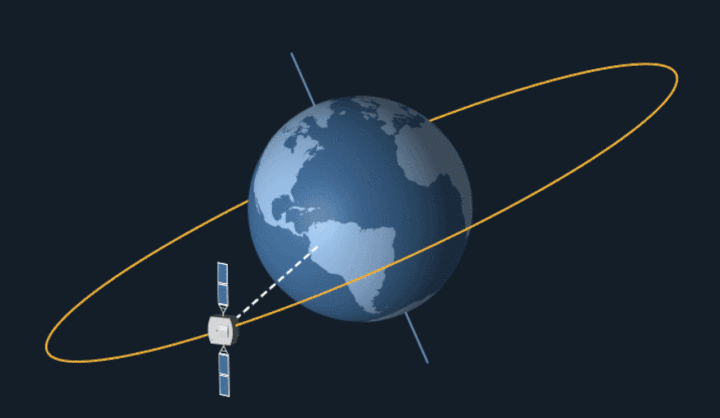## 引言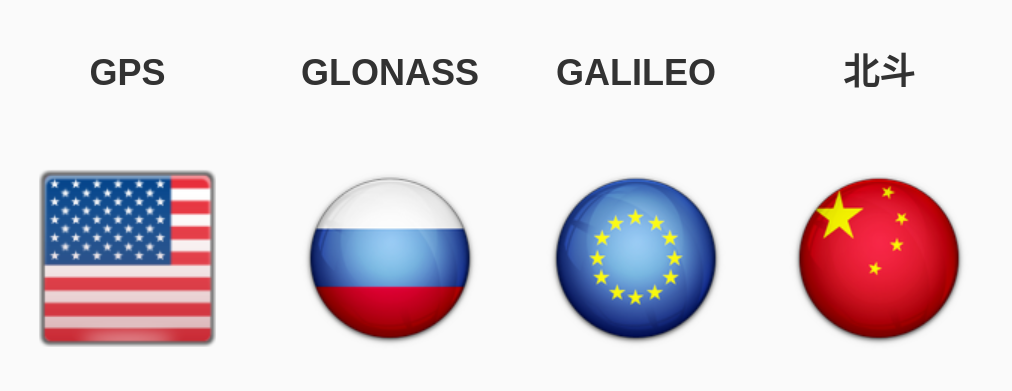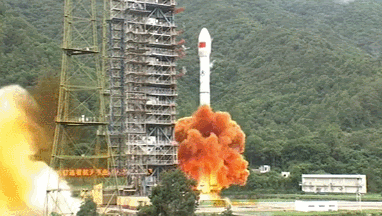• 第一阶段，2000年年底，发射2颗北斗一号卫星，建成北斗一号系统，向中国提供服务；
• 第二阶段，2007年-2012年，发射14颗北斗二号卫星，建成北斗二号系统，向亚太地区提供服务；
• 第三阶段，计划在2020年前后，发射30余颗北斗三号卫星，建成北斗三号系统，即北斗全球系统，向全球提供服务。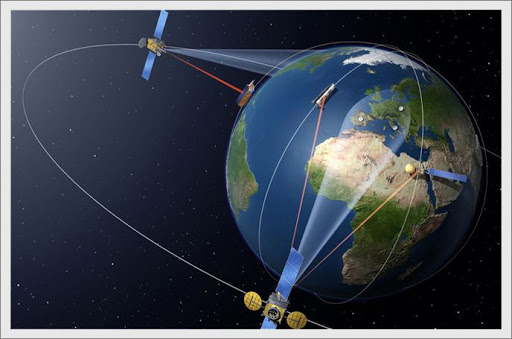## 计算

• 导航卫星：发射出包含时刻信息（t）和轨道信息（x, y, z）的无线电波；
• 监控站：负责监测卫星状态，确认卫星轨道并修正卫星时刻偏差；
• 接收器：接收卫星发射出来的无线电波。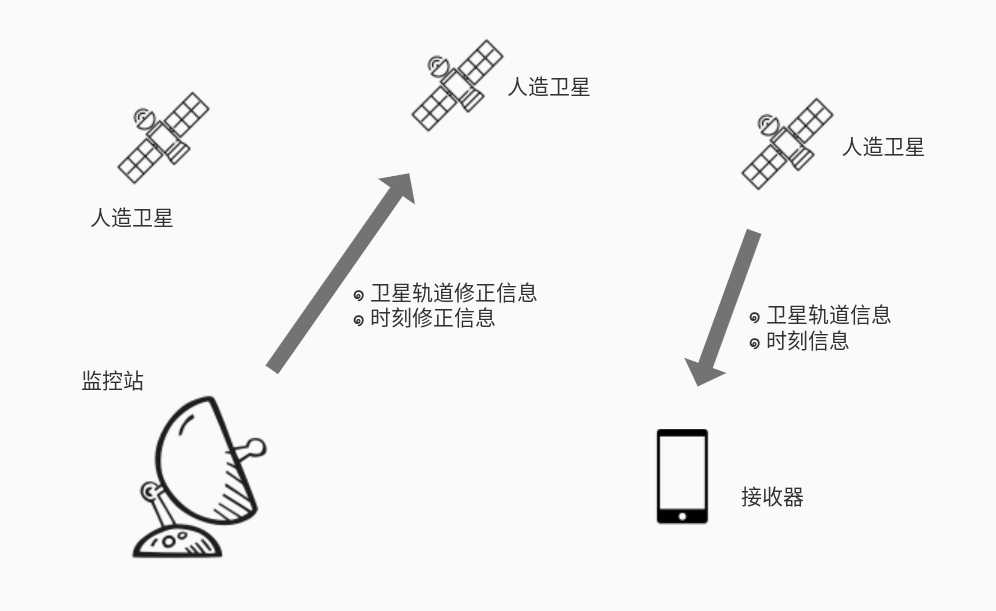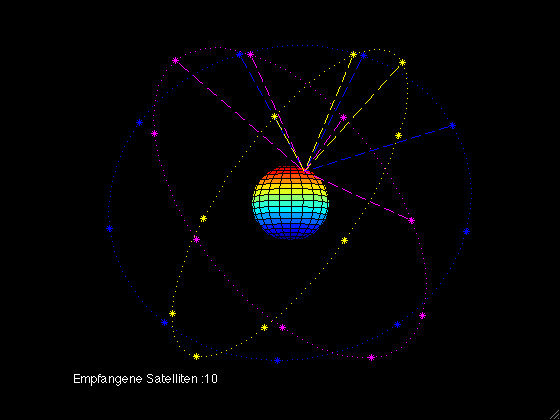$$AO^2 = {((T_a - T) \times c)}^2 = (X_a - X_o)^2 + (Y_a - Y_o)^2 + (Z_a - Z_o)^2$$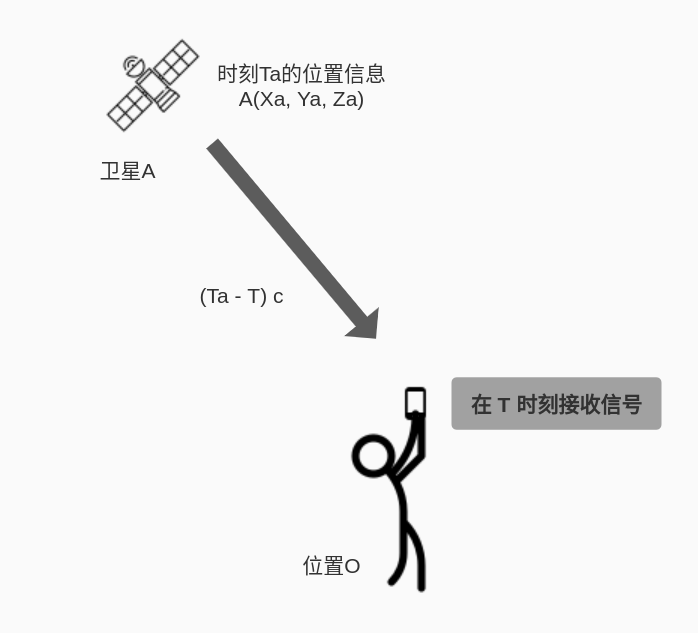$$AO^2 = {((T_a - T) \times c)}^2 = (X_a - X_o)^2 + (Y_a - Y_o)^2 + (Z_a - Z_o)^2$$

$$BO^2 = {((T_b - T) \times c)}^2 = (X_b - X_o)^2 + (Y_b - Y_o)^2 + (Z_b - Z_o)^2$$

$$CO^2 = {((T_c - T) \times c)}^2 = (X_c - X_o)^2 + (Y_c - Y_o)^2 + (Z_c - Z_o)^2$$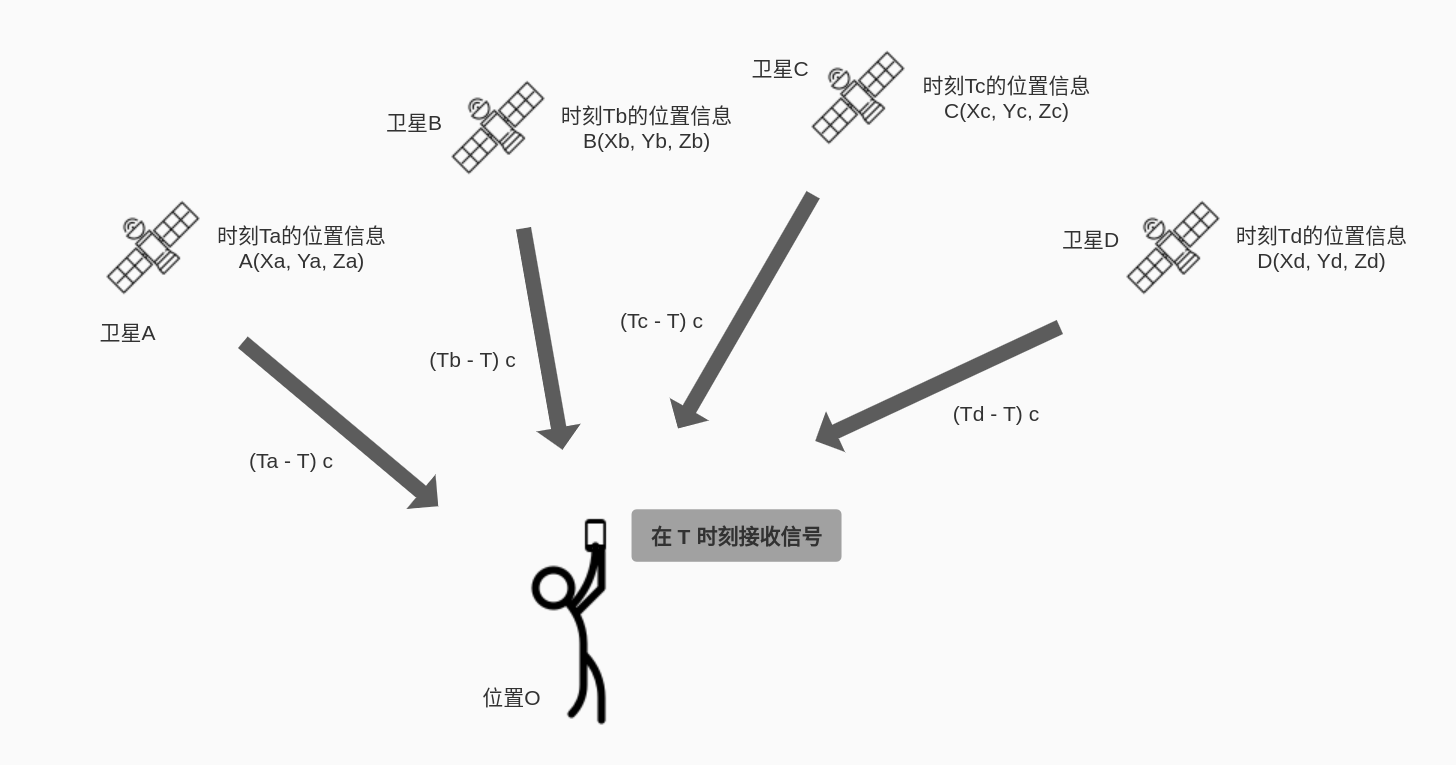$${((T_a - (T - \delta) \times c)}^2 = (X_a - X_o)^2 + (Y_a - Y_o)^2 + (Z_a - Z_o)^2$$

$${((T_b - (T - \delta) \times c)}^2 = (X_b - X_o)^2 + (Y_b - Y_o)^2 + (Z_b - Z_o)^2$$

$${((T_c - (T - \delta) \times c)}^2 = (X_c - X_o)^2 + (Y_c - Y_o)^2 + (Z_c - Z_o)^2$$

$${((T_d - (T - \delta) \times c)}^2 = (X_d - X_o)^2 + (Y_d - Y_o)^2 + (Z_d - Z_o)^2$$